# Team:BIT-China/modeling.html

MODELING | BIT-CHINA IGEM2013

# Overview

Why modelling?

Computational and mathematical models are useful and effective tools for understanding biology. Molecular biology experiments in vitro are very expensive and time consuming, building models of biological processes as a preliminary step helps to circumvent some of the drawbacks of performing hypothesis-testing in the wet lab. Using models could provide a faster and cheaper shortcut for biologists to gain a better understanding of the newly engineered system.Particularly with the extent of synthetic biology, many of the biological systems that are being researched could not be found in nature because they are genetically engineered, so their behaviors are unknown and need to be characterized.

Gene Regulatory Network

A gene regulatory network (GRN) is a collection of DNA segments in a cell which interact with each other indirectly (through their RNA and protein expression products) and with other substances in the cell, thereby governing the expression levels of mRNA and proteins. Some proteins serve only to activate or repress other genes, and these are the transcription factors that are the main players in regulatory networks or cascades. By binding to the promoter region at the start of other genes they turn them on or turn them off, initiating the production of another protein, and so on.

GRN of I’M HeRE

For one green world, an Intelligent Microbial Heat Regulating Engine (I’M HeRE), as shown in figure 1, was designed to satisfy our needs described in the project. The engine consists of 2 systems namely the heat-resistant system and the quorum-control system.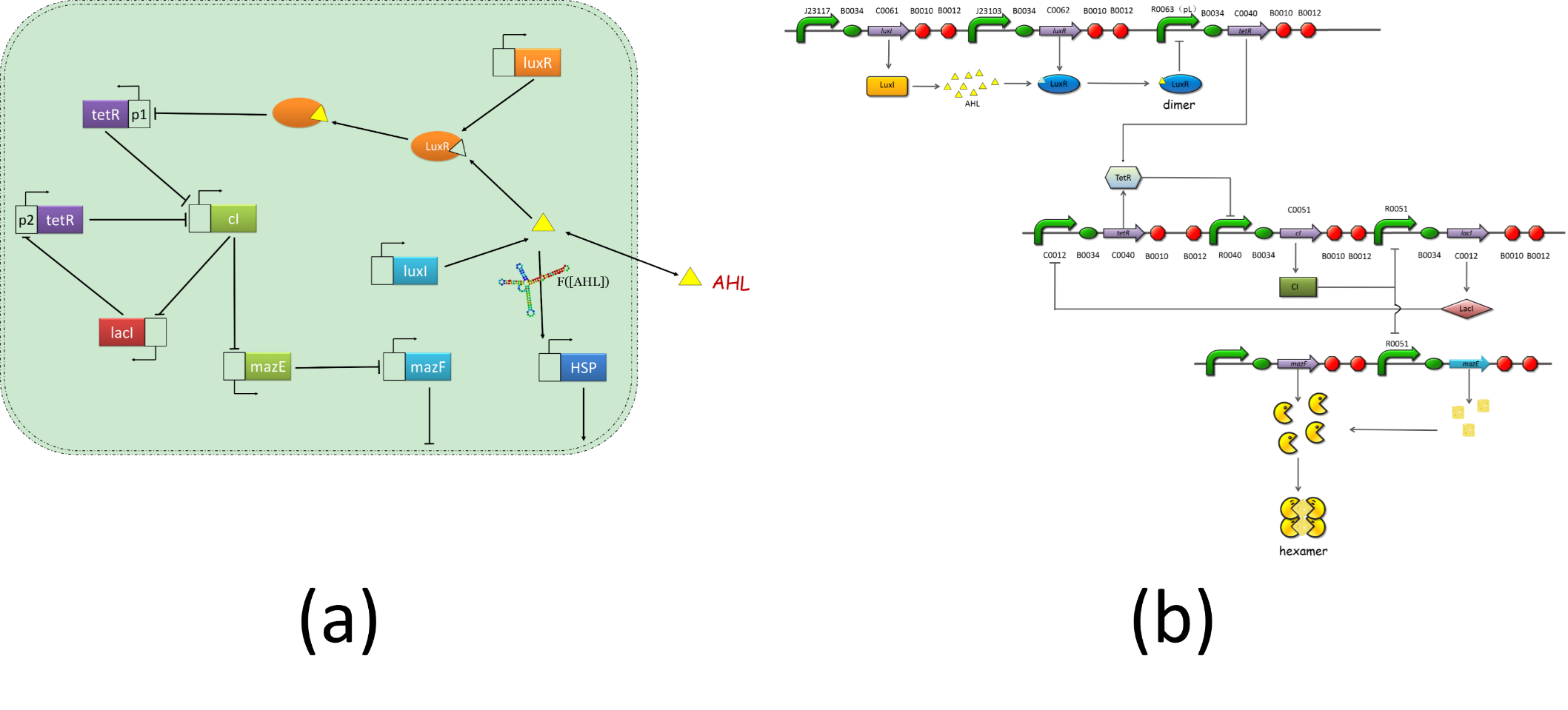Fig.1 The gene regulatory network of the intelligent microbial heat regulating . (a) shows the regulatory relationship of these genes. (b) is the detailed diagram of the GRN of the quorum control system.

When the number of cells increased to a certain extent, the gene regulatory network was started to control the number of live cells.

Method description

Currently, there are a variety of models, such as the directed graph model, Bayesian network model, Boolean network model, ODE models and stochastic differential equations models, could be applied to the analysis, modeling and simulation of gene regulatory networks.

ODE models implicitly assumes that the underlying quantities, i.e., concentrations or molecule numbers, vary in a continuous and deterministic fashion. However, at the molecular level these assumptions are mere approximations which might not accurately reflect the underlying dynamics, especially when the number of molecules involved is "small", such as is the case for transcription factors, which, in certain circumstances, can be expressed at low levels, i.e., a few tens of molecules, or for chromosomal DNA, for which a single copy exists in the cell.

Intrinsic stochasticity at the molecular level may not be neglected any more when small numbers of molecules are involved (since in this case the "averaging" out of stochastic effects due to the application of the law of large numbers does not hold any more). Therefore, stochastic models have to be considered.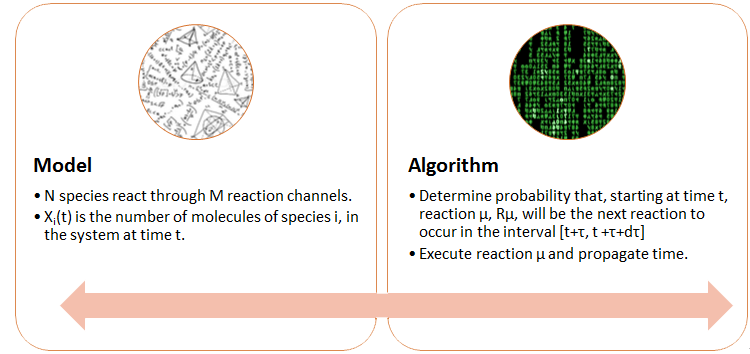The system may contain rare, discrete, but critical events and continuously occurring deterministic or stochastic transitions.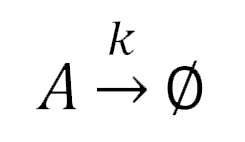• A is the chemical species of interest, and the number of molecules of chemical species A at time t is simply denoted as A(t)
• The symbol φ denotes chemical species which are of no further interest in what follows.
• k is the rate constant of the reaction and is defined so that k*dt gives the probability that a randomly chosen molecule of chemical species A reacts (is degraded) during the time interval [t, t + dt)
• the probability that r < A(t)k∆t is equal to A(t)k∆t, since r is a random number uniformly distributed in the interval (0, 1)
• A(t)*kdt gives the probability that exactly one reaction occurs during the infinitesimal time interval [t, t+dt)

The goal is to compute the number of molecules A(t) for times t > 0.With k = 0.1, protein (0) = 20 and ∆t = 0.005.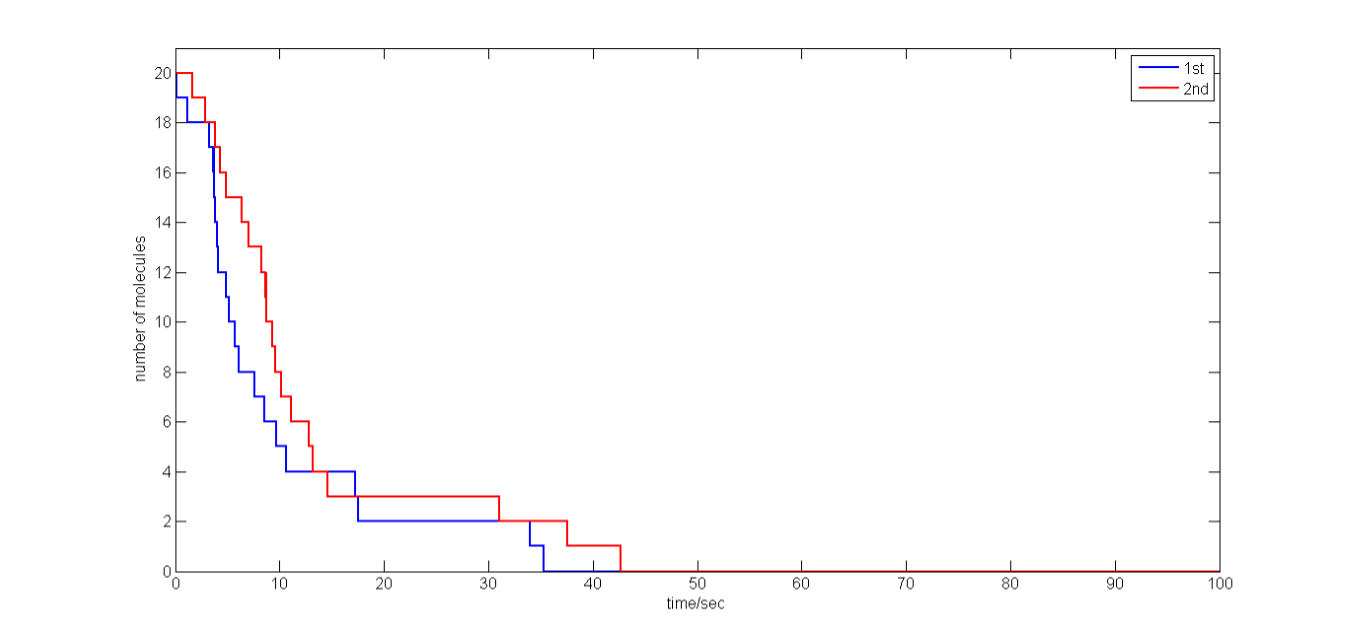Fig.2 Stochastic simulation of protein degradation for k = 0.1, protein (0) = 20 and ∆t = 0.005. Number of molecules of proteins as a function of time for two realizations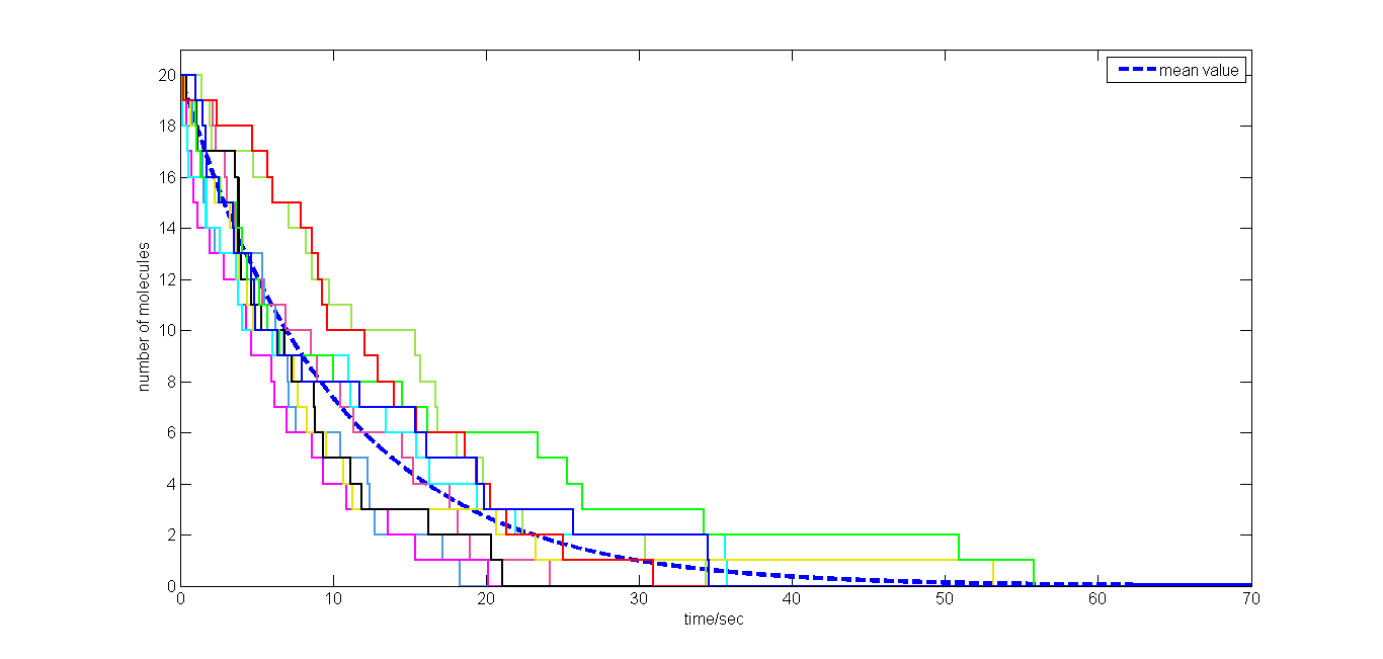Fig.3 Stochastic simulation of protein degradation for k = 0.1, protein (0) = 20 and ∆t = 0.005. Number of molecules of proteins as a function of time for ten realizations. The blue dash line shows the mean value of the stochastic results.

• Stochastic simulation of production and degradationWith Protein (0) = 0, k1 =0.1 and k2= 1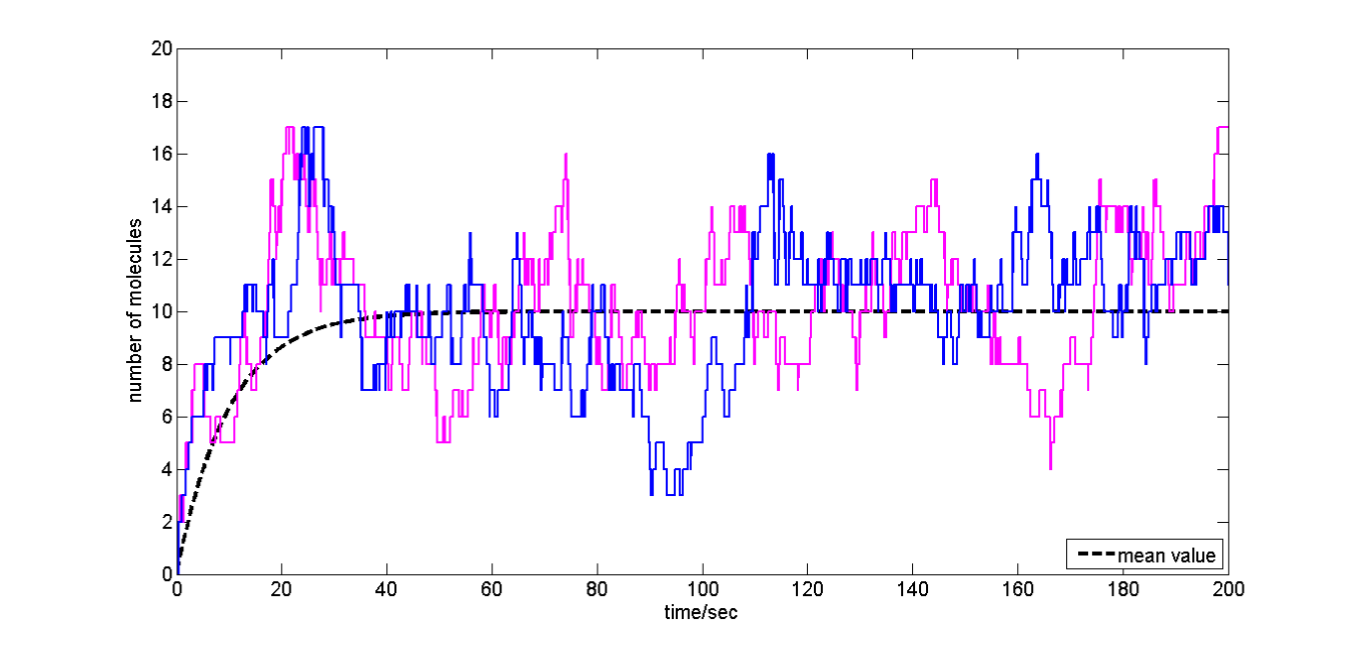Fig.4 two realizations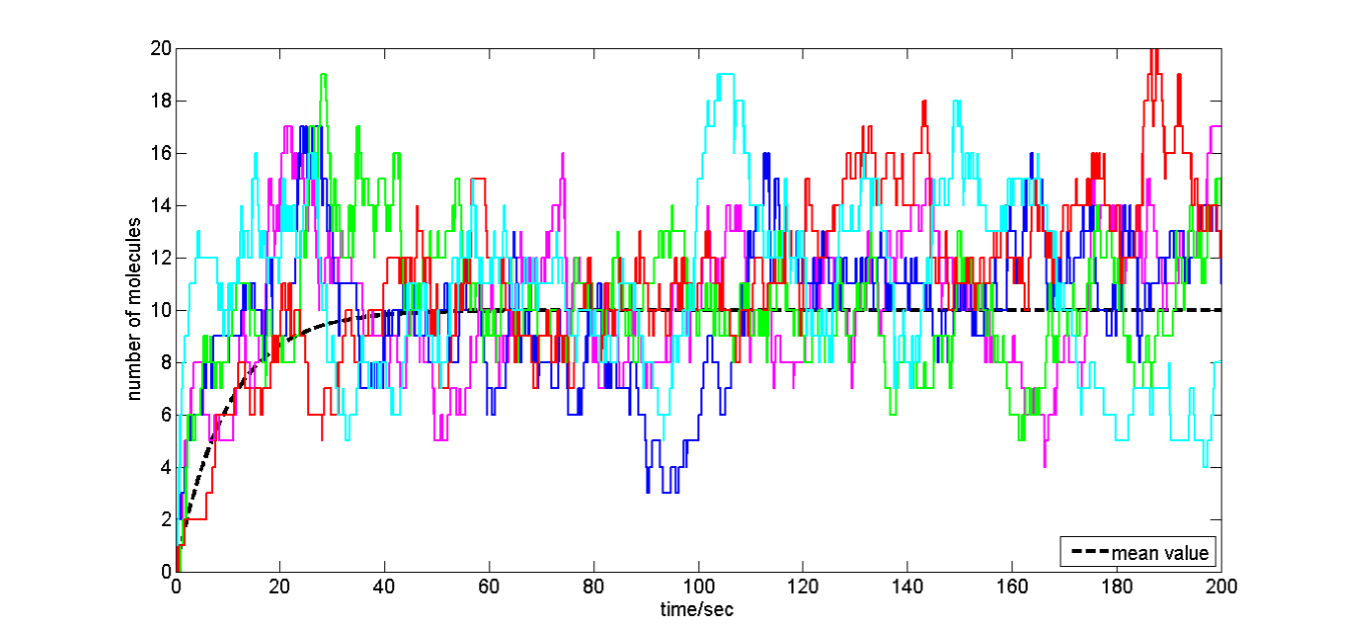Fig. 5 ten realizations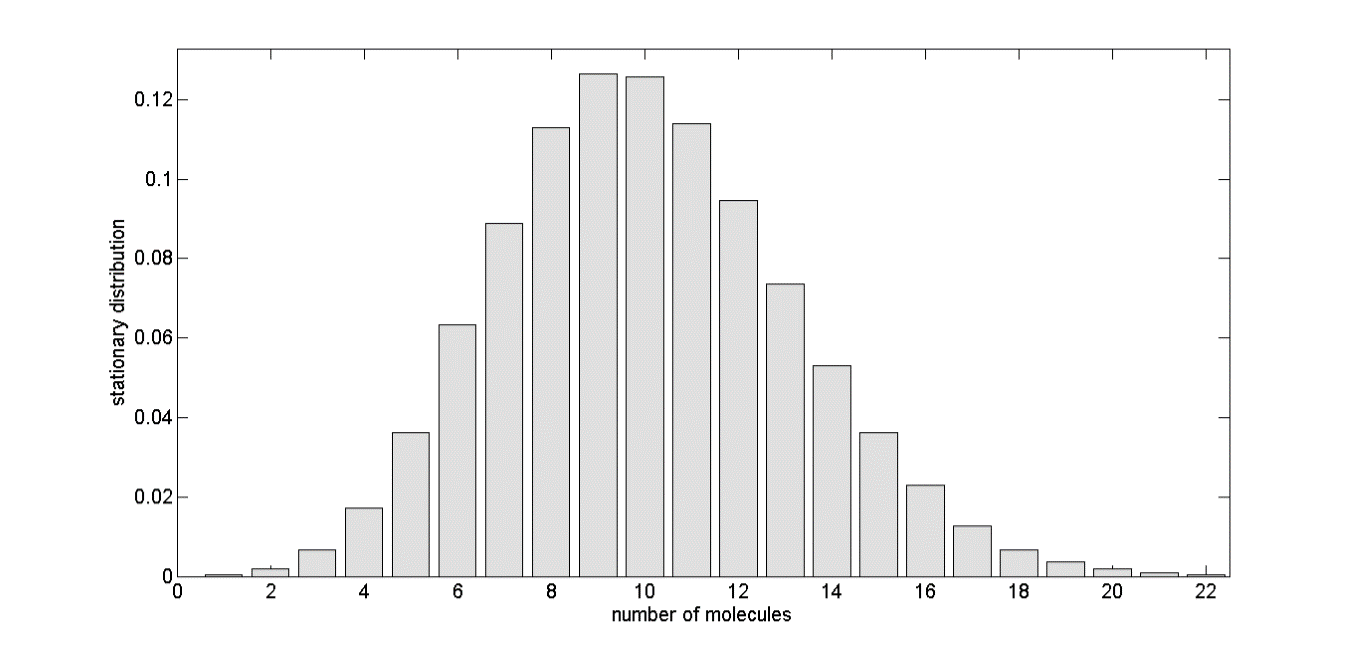Fig.6 stationary distribution of the molecules, it’s analyzed to be poison disribution

• Stochastic simulation of dimer formationWith A(0)=0, B(0)=0, k1=10-3,k2=10-2, k3=1.2 and k4=1.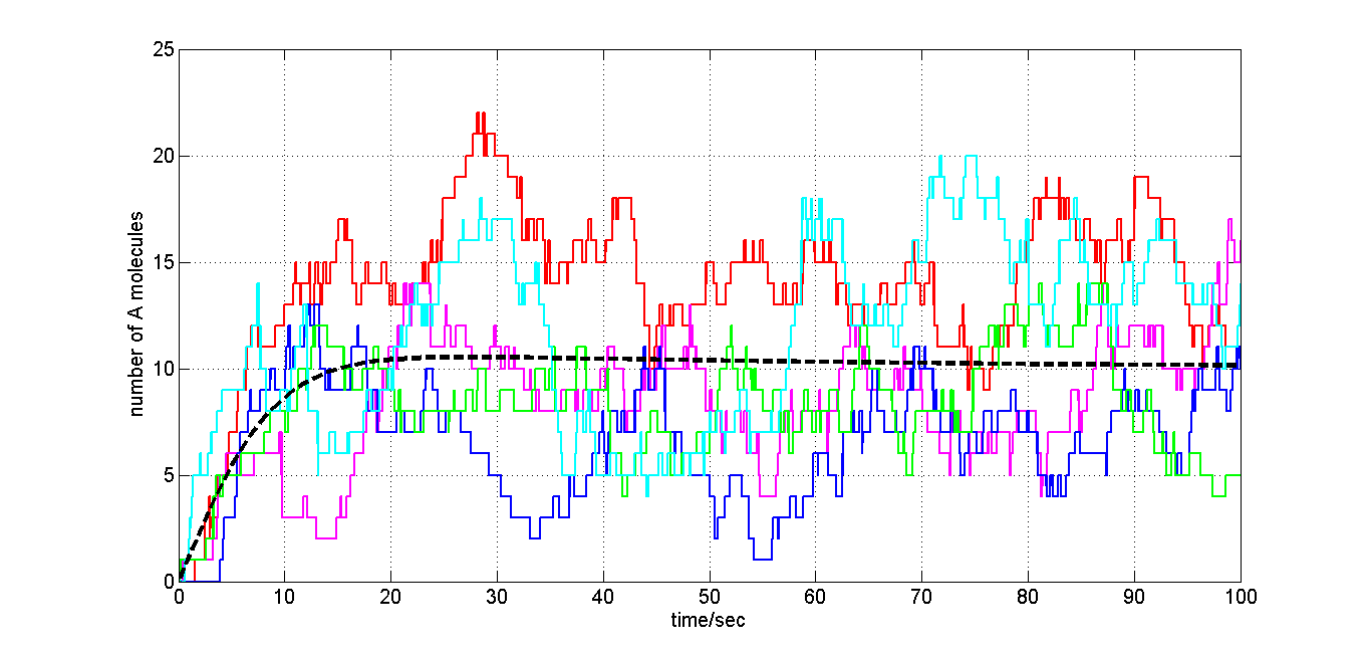Fig.7 number of A molecules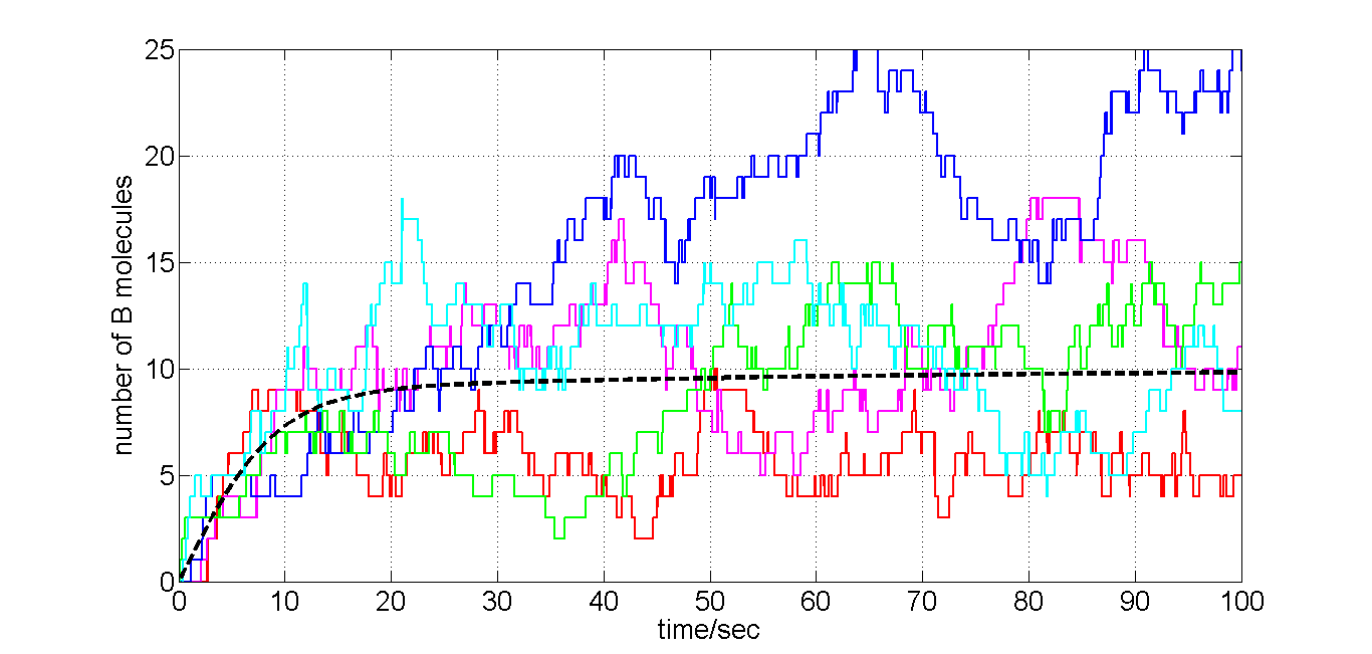Fig.8 number of B molecules

Modelling the gene regulatory network

The GRN is too complicated to conducted biological experiments. Thus dynamic simulation of the GRN by mathematical modelling is particularly important. Before the wet experiments were conducted, mathematical models were built to predict the behavior of our heat regulating engine. Such bottom-up design of synthetic gene circuit will not only speed up the biology research, but provide us a valuable insight into the system as well. We want to know:

• In a particular state which genes were expressed?
• What’s the influence of the gene product on cell physiological activities?
• To provide a guidance on the design of experiments by further analysis of the dynamic behavior of gene regulatory networks.

# Noise in the cell

The cellular environment is abuzz with noise. A key source of this “intrinsic” noise is the randomness that characterizes the motion of cellular constituents at the molecular level. Cellular noise not only results in random ﬂuctuations (over time) within individual cells, but it is also a source of phenotypic variability among clonal cellular populations. Researchers are just now beginning to understand that the richness of stochastic phenomena in biology depends directly upon the interactions of dynamics and noise and upon the mechanisms through which these interactions occur.

# Quorum Control system

The quorum control system was composed of 3 device.

Goals:

• We want a better understanding of the process of gene regulation by analyzing the dynamical properties of the gene regulatory networks.
• Assumption:

• In this project, the simulation was performed in only a single cell.Bacteria communicate with each other using chemical signaling molecules. The process of producing, releasing, detecting, and responding to the signaling molecules in bacteria is referred to as quorum sensing. Quorum sensing enables bacteria to obtain information from their environment and alter their behavior in a population size that is scaled in response to changes the number of bacteria present in their community. Thus, this method of intercellular communication allows populations of bacteria to work together, in contrast to the traditional unicellular view of prokaryotes.

In general, quorum-sensing bacteria produce and release signaling molecules into their environments which are called auto-inducers. The concentrations of auto-inducers increase in the bacteria environment as the bacteria population density grows. The bacteria detect the accumulation of a minimal threshold concentration of the auto-inducer and regulate their gene expression in a population-wide scale in response. As a result, using these signaling systems, all the bacteria in a community alter their behavior at the same time in response to an external signal, and therefore function similar to a multicellular organism.(indexed from Wikipedia)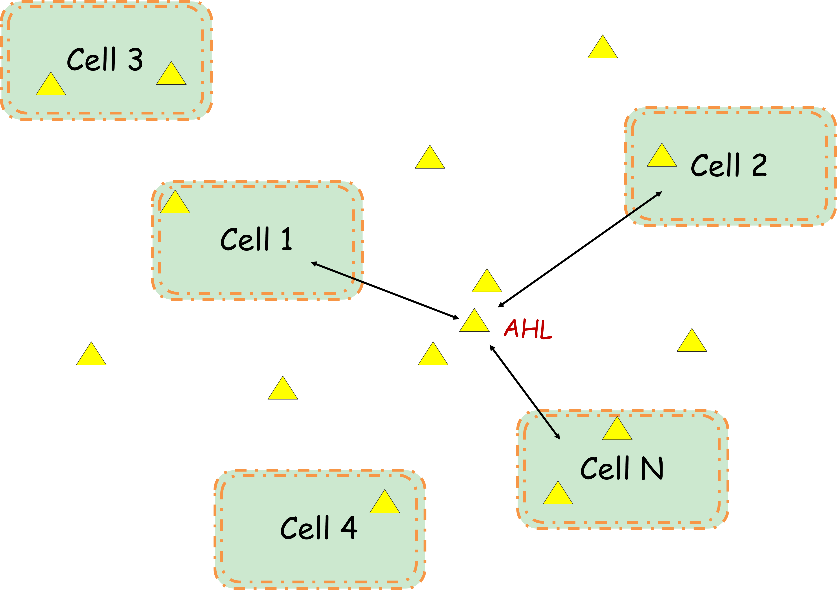Many different quorum-sensing systems have been discovered. Some of them are extremely specific for a particular species and some of them are common in all bacteria. In this work, we sought to establish a synthetic auto-inducer (AHL) signaling system.

It is assumed that AHL was produced and degraded at a certain rate to maintain a certain concentration in a single cell. That is to say the number of AHL molecules within a single cell is constant. Therefore, there is a linear relationship between the AHL molecule concentration and cell concentration. The regulating engine was started when the number of AHL molecules reaches a certain threshold, or when the number of cells reaches a certain threshold.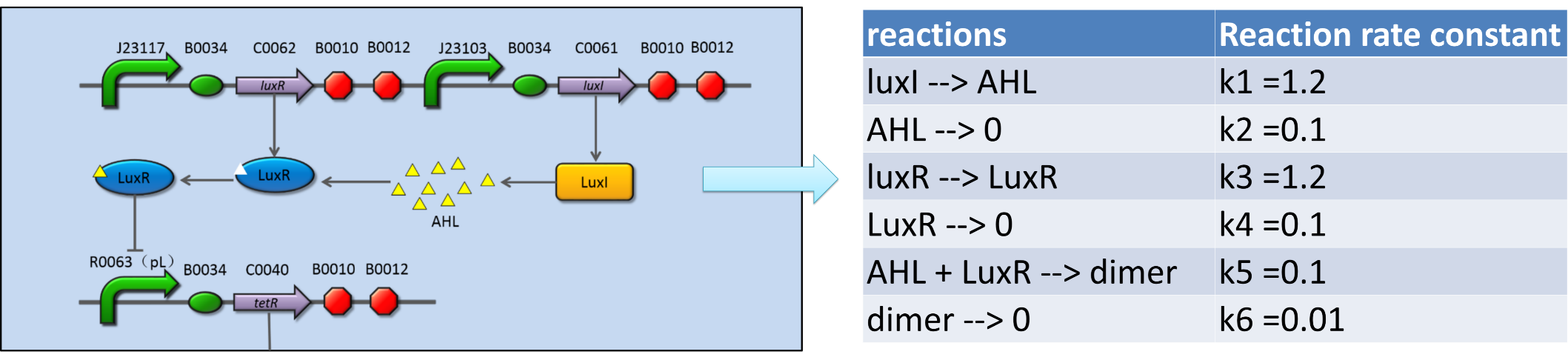Fig.9 the GRN of the quorum sensing device. The right table shows the chemical reactions of the GRN.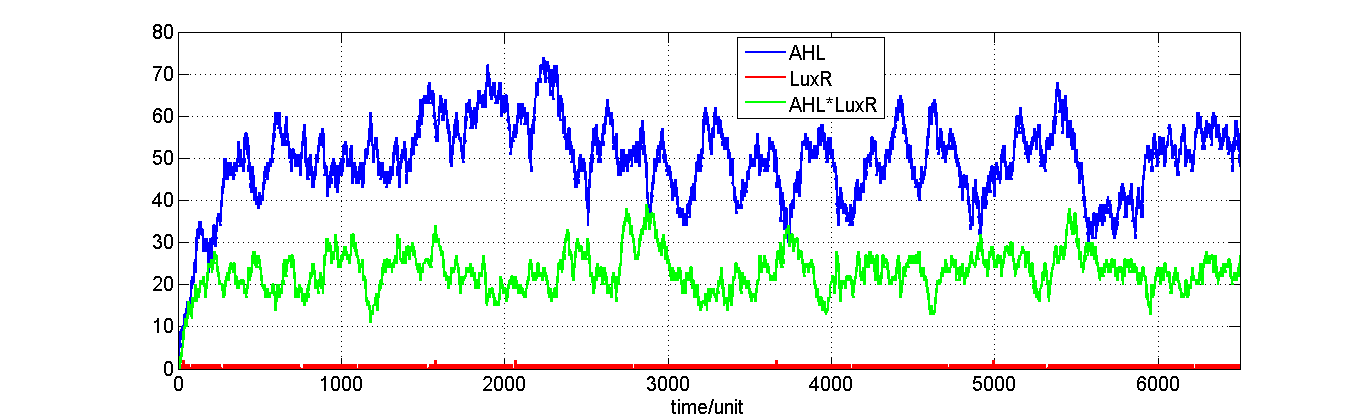Fig.10 stochastic simulation of the GRN in a single cell

As we could see from the simulation result in fig.10. AHL was produced and degraded at a certain rate to maintain a certain concentration in a single cell. A AHL*LuxR complex was formed once LuxR was produced. Thus the concentration of LuxR was low.The oscillator is a synthetic biology construct composed of three genes which mutually repress each other in sequence according to a ring structure.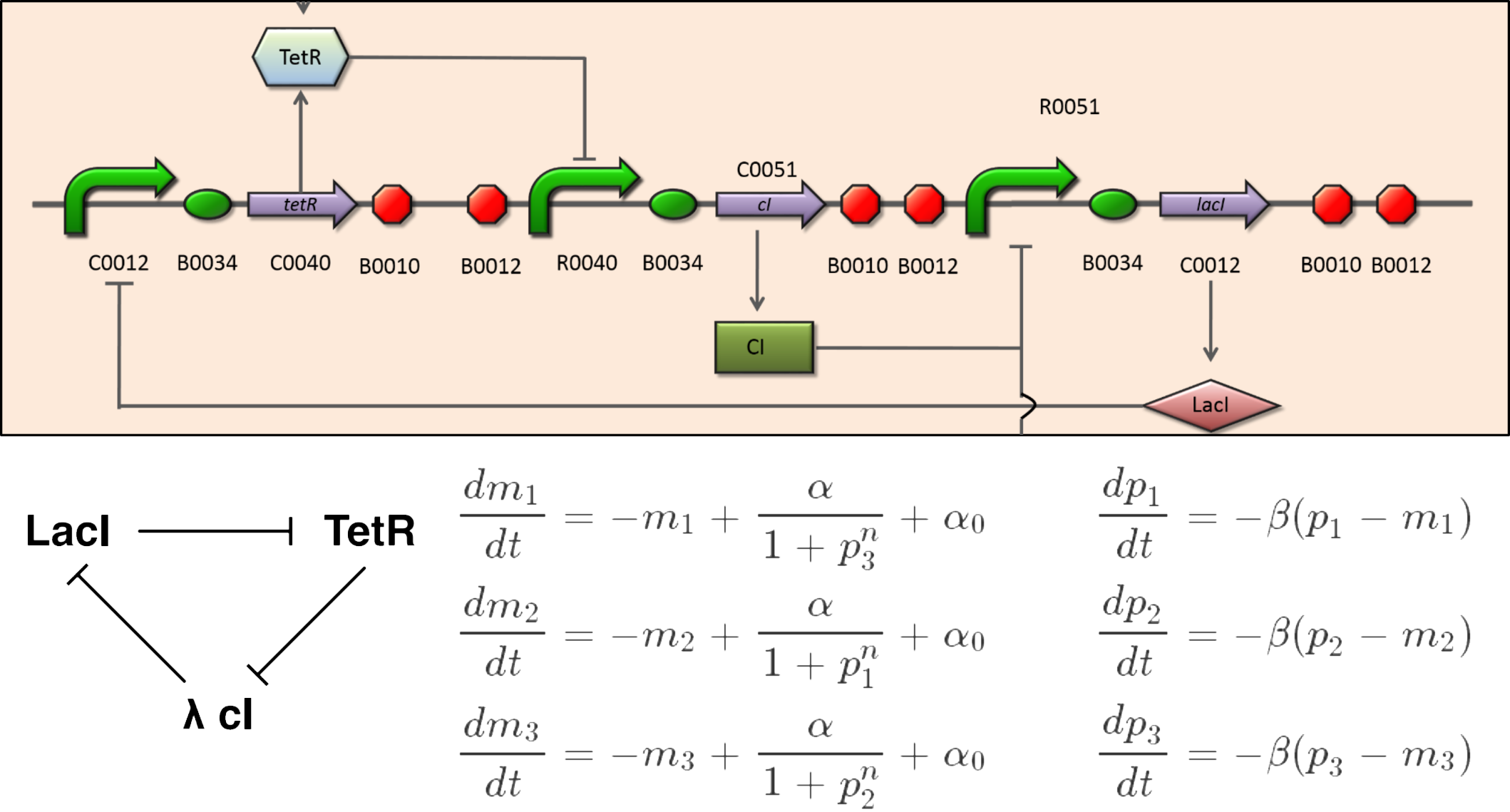Fig.11 detailed GRN of the oscillator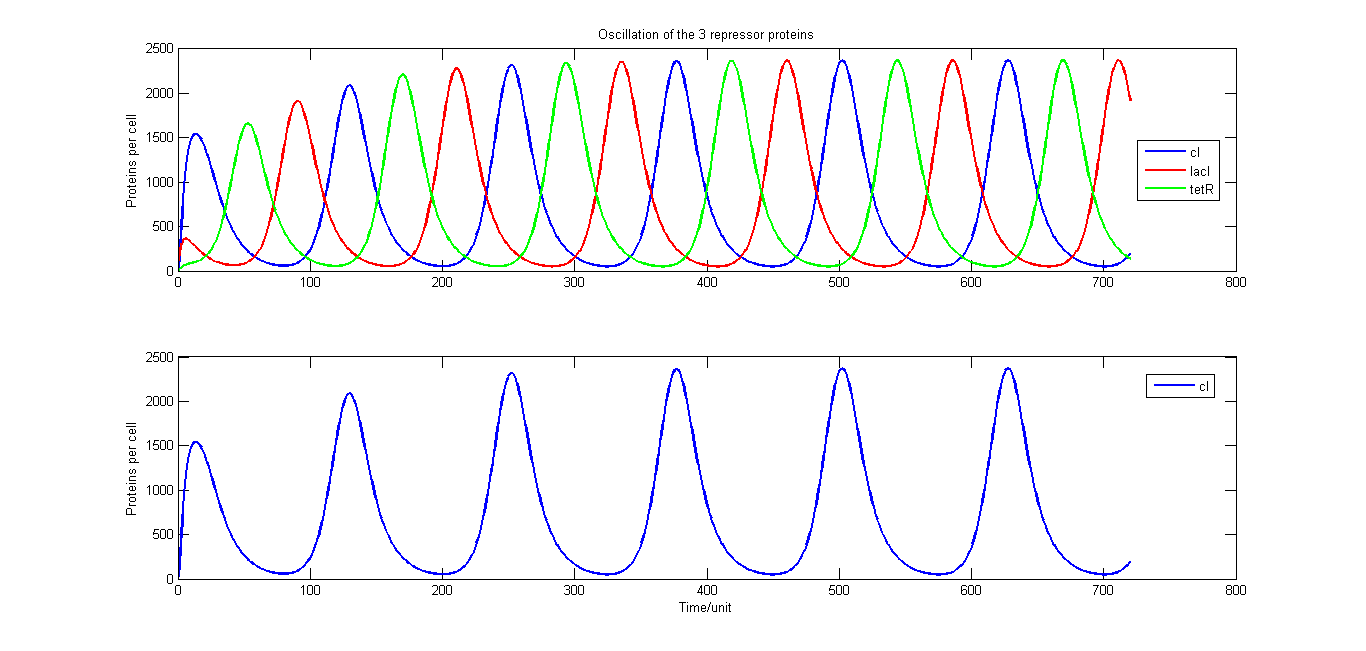Fig.12 ODE simulation of the oscillator

An experiment showing the oscillator was shown here http://www.youtube.com/watch?v=HFwSnqYC5LA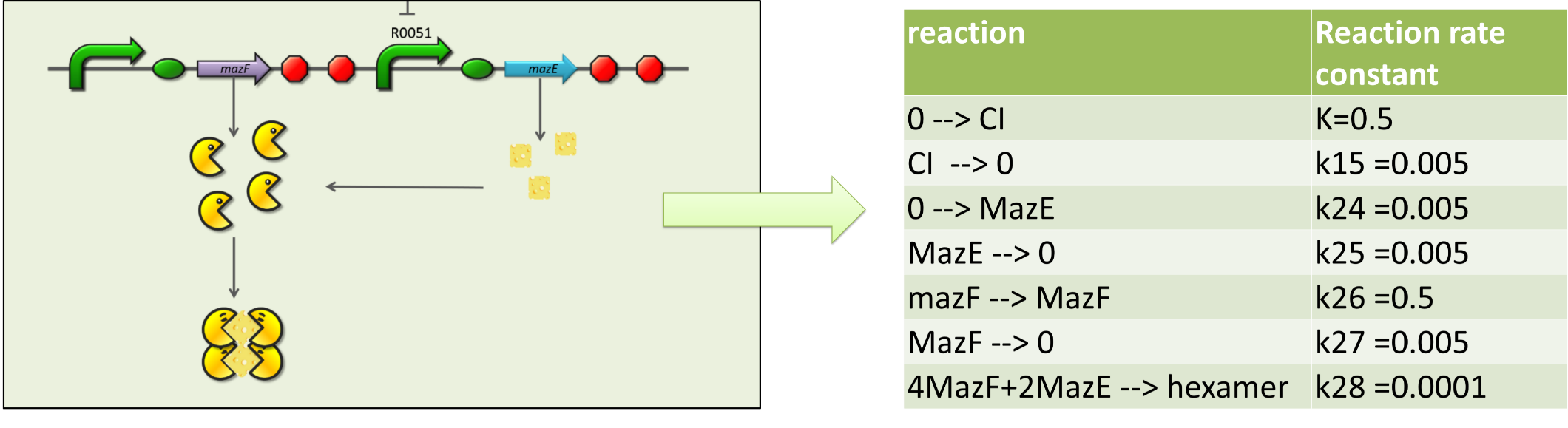Fig.13 the GRN of the PCD device. The right table shows the chemical reactions of the GRN.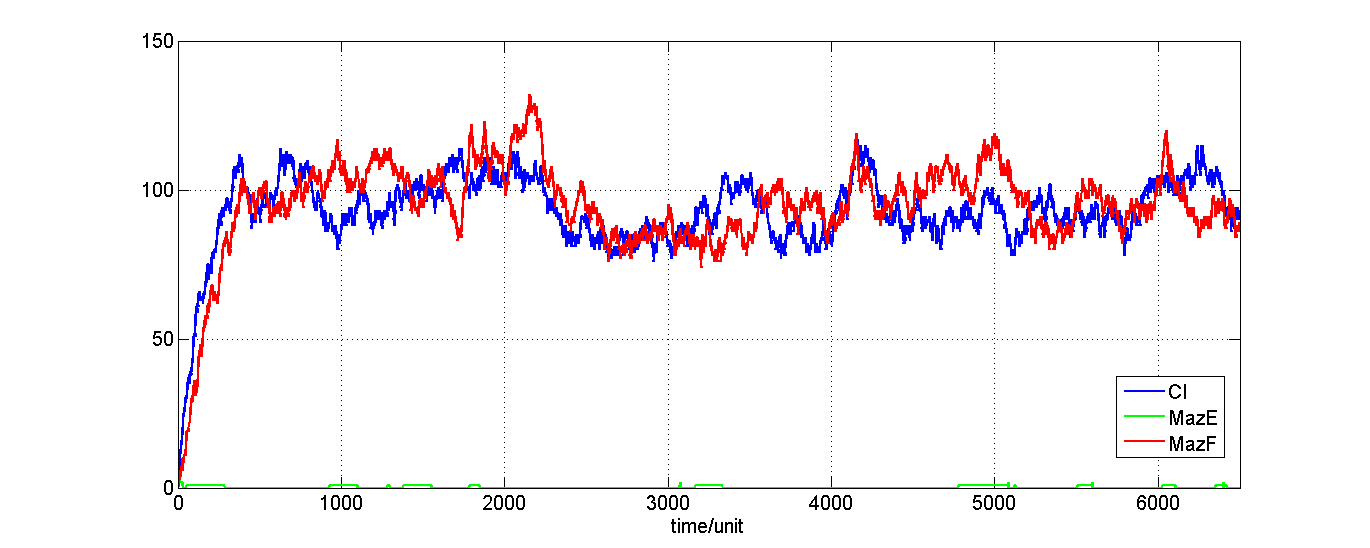Fig.14 Stochastic simulation of the PCD

# Heat Resistant systemThe heat resistant system was made up by a RNA thermometer and a heat shock protein expression device.

Questions

• What’s the shape of the growth curve under normal growth condition?
• What the impact if the lethal effect is considered?
• What is the time to turn on the RNA temperature switch?
• What happened if the cooling system was removed?
• Build the normal cell growth model
• Build the growth model with the lethal effect considered
• Build a model which can be used to predict the temperature change tendency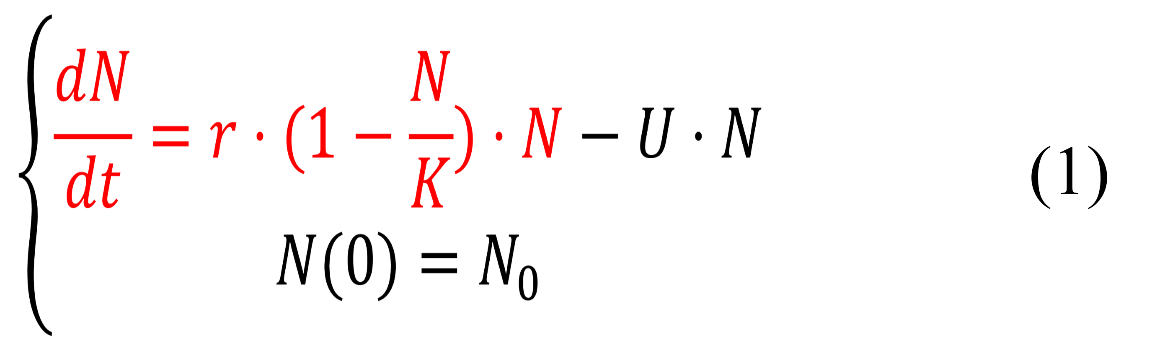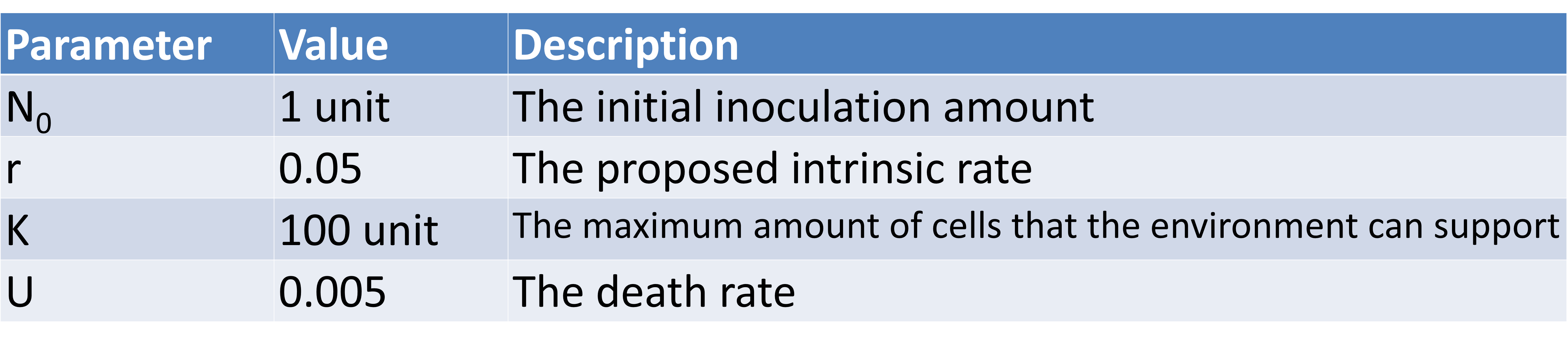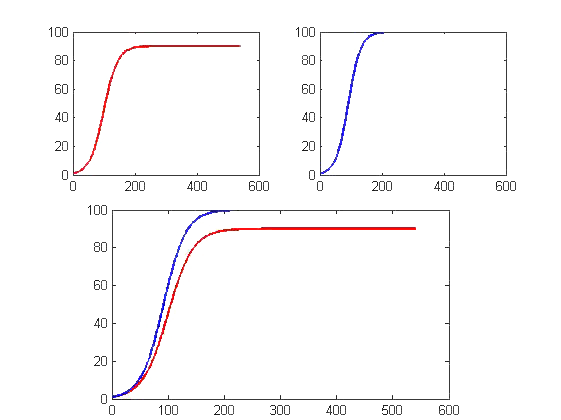Fig. 14 the red curve shows the result with the lethal effect considered, the blue curve shows the normal logistic growth result.

The initial inoculation amount of this model is 1 unit. The proposed intrinsic rate is 0.05. And we set the maximum number of cells that the environment can support is 100 units. As can be seen from the simulation , from 0 to 200 units of time, the number of cells are in a growth state in both cases, no lethal effect is greater than the growth rate of cells in the presence simulation lethal effect of simulations; after 200 units of time, two kinds of simulations have tended to steady state, the growth of non-lethal effects of simulated results of the final number of the environment can accommodate the largest state, while the presence of the number of lethal effect of significantly less than the maximum number of steady-state simulation results is about 90 units.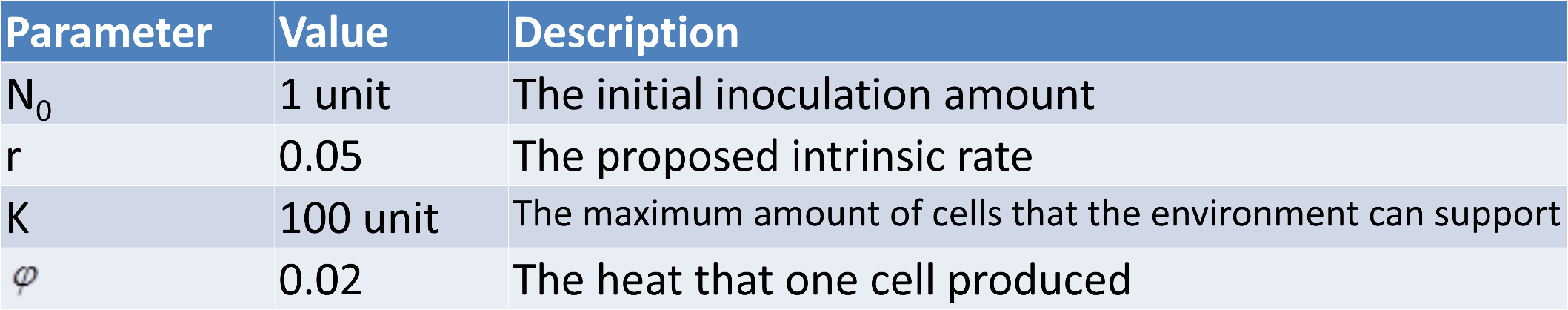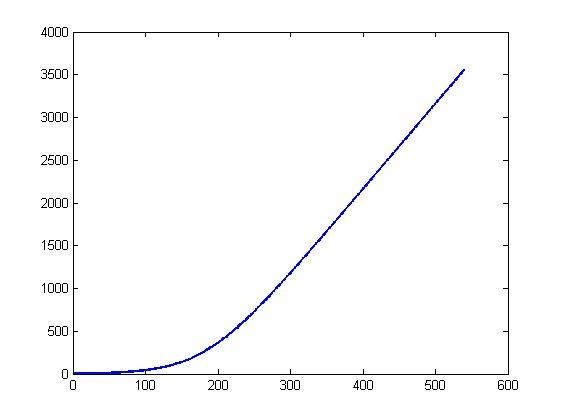Fig.15 time evolution of heat accumulated in the fermenter

We could get the information that the heat in the fermenter will accumulate over time without a cooling system from the above figure. Once the cooling system was turned on, a steady state could be achieved as shown in the figure above.

# Reference

 Esmaeili A, Yazdanbod I, Jacob C. A Model of the Quorum Sensing System in Genetically Engineered E. coli Using Membrane Computing[J]. International Genetically Engineered Machine (iGEM) competition, 2009.

 Paustian K, Pankhurst C E, Doube B M, et al. Modelling soil biology and biochemical processes for sustainable agriculture research[J]. Soil biota: management in sustainable farming systems., 1994: 182-193.

 Erban R, Chapman J, Maini P. A practical guide to stochastic simulations of reaction-diffusion processes[J]. arXiv preprint arXiv:0704.1908, 2007.

 Fournier T. Stochastic models of a self regulated gene network[D]. , 2008.

 Johansen S H. Modelling and Analysis of a Synthetic Bistable Genetic Switch[D]. Norwegian University of Science and Technology, 2011.

 Scott M. Tutorial: Genetic circuits and noise[J].